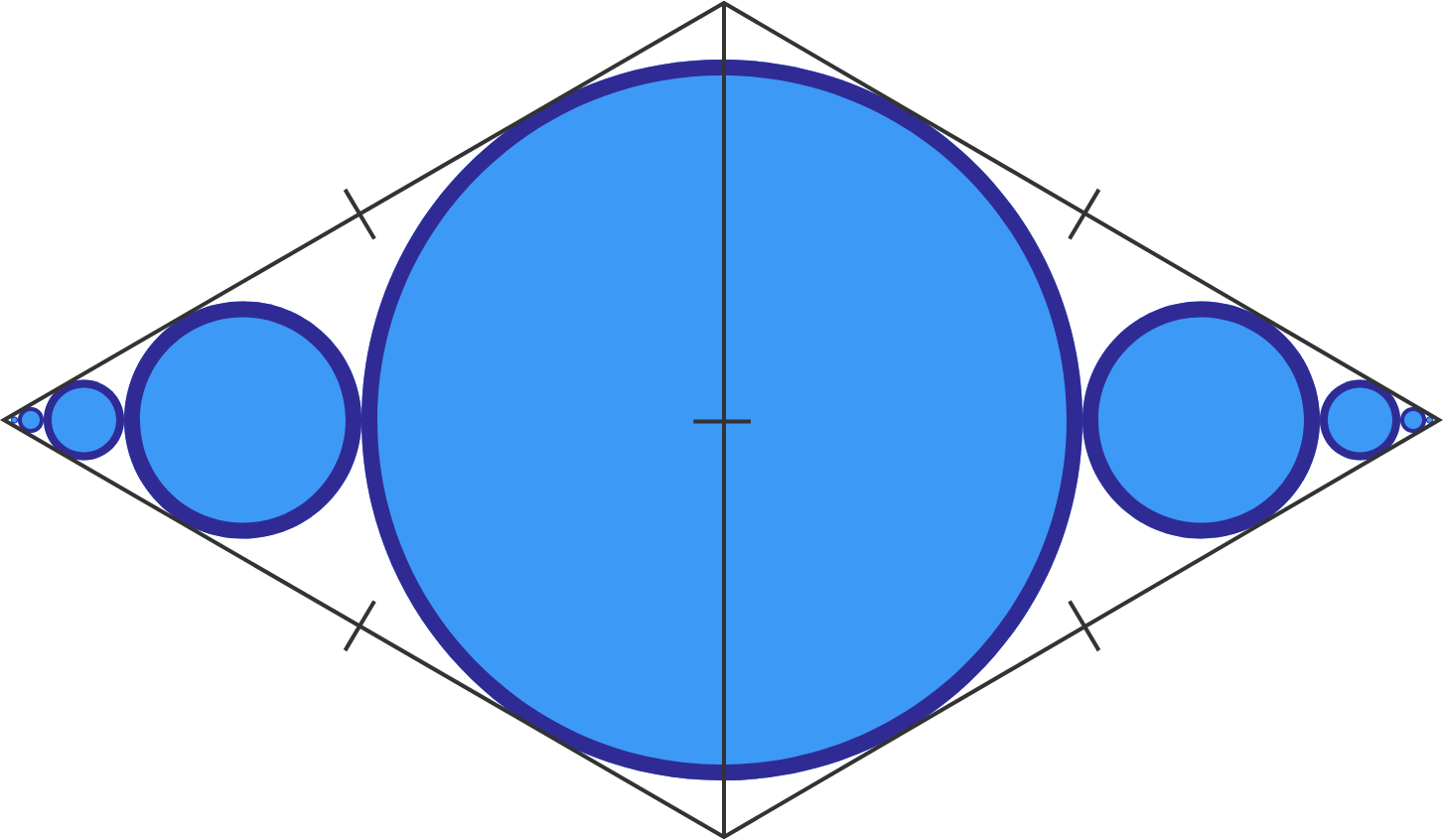# Circles And Circles And Circles

Geometry Level 5Two equilateral triangles are joined to create a rhombus. A circle is inscribed so that its center lies on the midpoint of the triangle sides that touch. Two more circles are inscribed, one on each side of the largest circle, such that they touch the sides of the triangle and the largest circle. This pattern repeats to infinity as shown.

Given that the radius of the largest circle is 1, find the sum of all circle areas, that is, the sum of the blue shaded region.

If the area can be expressed as $\dfrac{a \pi}{b}$, where $a$ and $b$ are coprime positive integers, input $a+b$ as your answer.

×

Problem Loading...

Note Loading...

Set Loading...